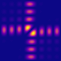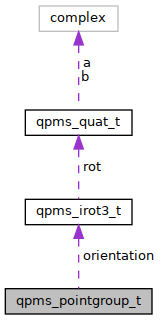QPMS Electromagnetic multiple scattering library and toolkit.
qpms_pointgroup_t Struct Reference

Full characterisation of a 3D point group. More...

#include <qpms_types.h>

Collaboration diagram for qpms_pointgroup_t:[legend]

## Data Fields

qpms_pointgroup_class c
Point group classification.

qpms_gmi_t n

qpms_irot3_t orientation
Transformation between this point group and the "canonical" point group of the same type. More...

## Detailed Description

Full characterisation of a 3D point group.

## ◆ n

 qpms_gmi_t qpms_pointgroup_t::n

Order of the rotational subgroup $$\mathrm{C_n}$$ of finite axial groups.

## ◆ orientation

 qpms_irot3_t qpms_pointgroup_t::orientation

Transformation between this point group and the "canonical" point group of the same type.

Each 3D point group of a given type (specified by the c and n members) has its isomorphous "canonical instance", typically with the main rotation axis identical to the z cartesian axis and a mirror plane (if applicable) orthogonal to the x cartesian axis.

If $$o$$ is a transformation specified by orientation, then an element $$g$$ of this group can be written as

$g = o g_\mathrm{can.} o^{-1}$

where $$g_\mathrm{can.}$$ is a corresponding element from the canonical instance.

CHECKME $$o$$ transforms the cartesian z axis to the main rotation axis of this group (if applicable).

TODO more detailed specification about the canonical instances and in which direction orientation goes.

The documentation for this struct was generated from the following file: